# 一、傅立叶级数的由来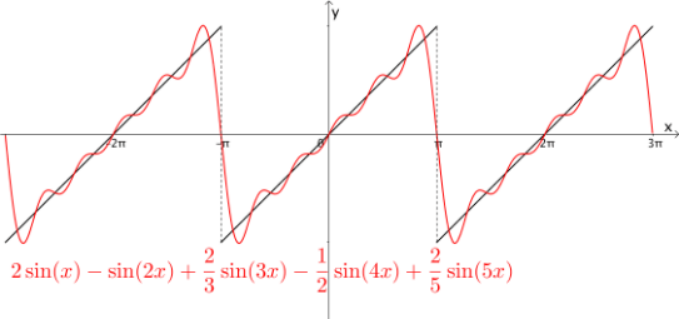# 二、傅立叶级数公式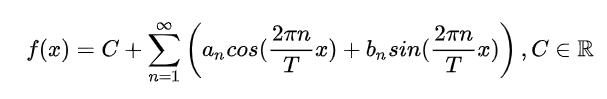1、常数项C，用来调整整体位置。
2、因为任意函数都可以分解为奇函数和偶函数之和，所以正弦、余弦函数是组成任意函数的基础，他们之间经过合理的加减组合，可以成为任意函数，必须有！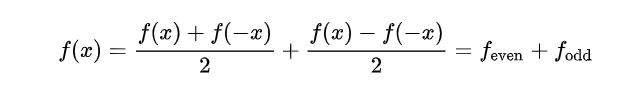3、调整振幅（振幅是正弦、余弦函数前边的系数），为了使其尽可能的逼近原函数。
4、正弦、余弦函数内的系数是为了让他们周期为T，即所有三角函数的和组成的函数要与他们要逼近函数的周期相同。

# 三、频域和时域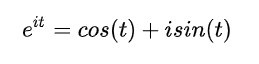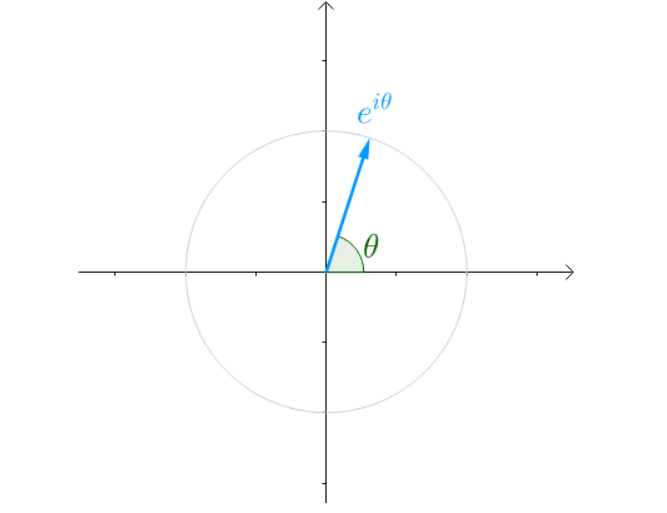即欧拉公式代表的就是单位圆上的点。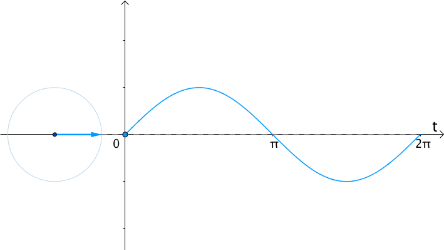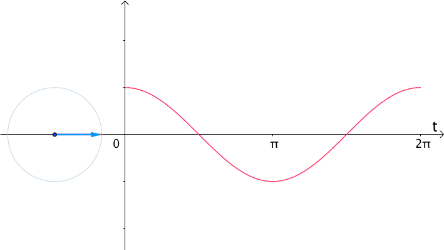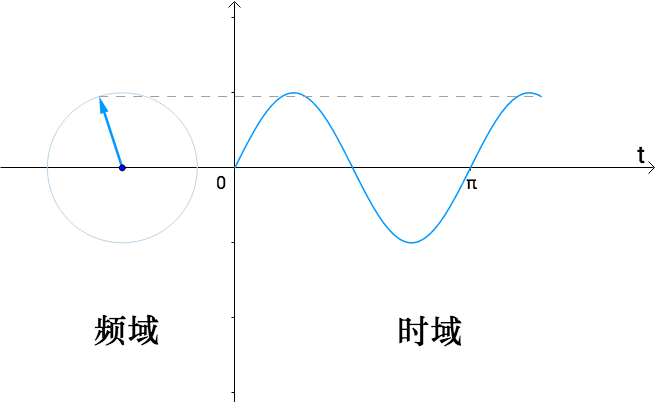# 四、傅立叶级数公式的进一步求解

## 4-1、抛砖引玉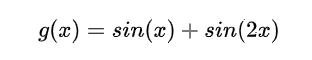e i t + e i 2 t = （ cos ⁡ ( t ) + cos ⁡ ( 2 t ) ） + i （ sin ⁡ ( t ) + sin ⁡ ( 2 t ) ） {\rm e}^{it}+{\rm e}^{i2t}=（\cos(t)+\cos(2t)）+i（ \sin(t)+\sin(2t)）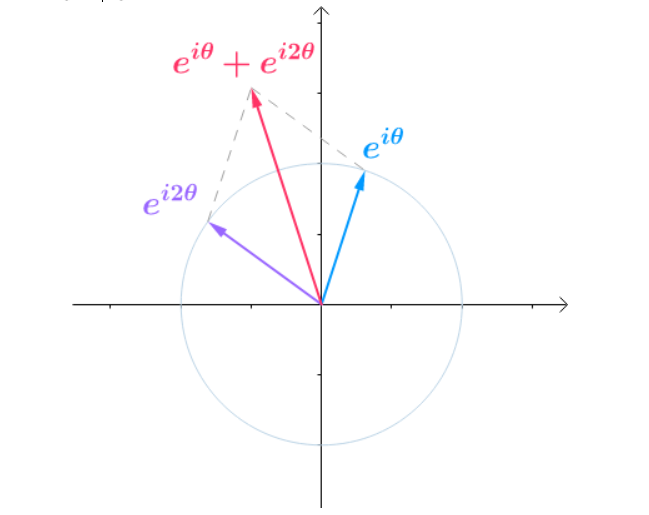G ( t ) = e i t + e i 2 t G(t)= {\rm e}^{it}+{\rm e}^{i2t}

w ⃗ = 2 u ⃗ + 3 v ⃗ \vec{w} = 2\vec{u}+ 3\vec{v}

u ⃗ ⋅ v ⃗ = 0 \vec{u} \cdot \vec{v} = 0

u ⃗ \vec{u} 的系数a可以计算为：
w ⃗ ⋅ u ⃗ u ⃗ ⋅ u ⃗ = 2 \frac{\vec{w} \cdot \vec{u}} {\vec{u} \cdot \vec{u}} = 2

v ⃗ \vec{v} 的系数b可以计算为：
w ⃗ ⋅ v ⃗ v ⃗ ⋅ v ⃗ = 3 \frac{\vec{w} \cdot \vec{v}} {\vec{v} \cdot \vec{v}} = 3

w ⃗ ⋅ u ⃗ = a ⋅ u ⃗ ⋅ u ⃗ \vec{w} \cdot \vec{u} = a \cdot \vec{u} \cdot \vec{u}
( 2 u ⃗ + 3 v ⃗ ) ⋅ u ⃗ = a ⋅ u ⃗ ⋅ u ⃗ (2\vec{u} +3\vec{v})\cdot \vec{u} = a \cdot \vec{u} \cdot \vec{u}

os：先记住这个结论就好了，不要问那么多为什么，我是个数学渣渣能推到这已经很不容易了，先糊弄过去。

os：同样的先记住这个结论。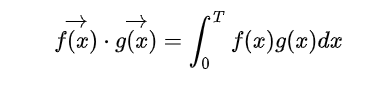## 4-2、傅立叶级数公式进化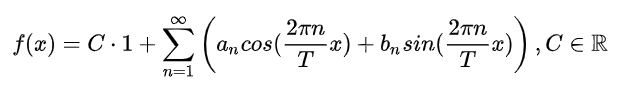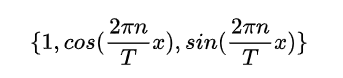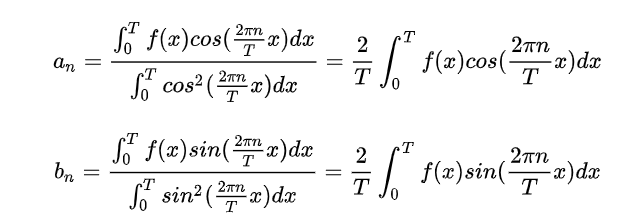C也可以通过点积来表示：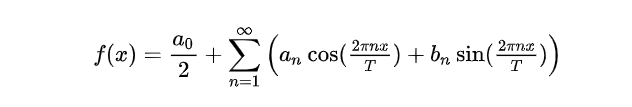# 总结

01-19786
03-14525

10-111万+
12-232万+
05-13128
11-287980
12-034859
07-05861
11-25355
03-22487
11-11793
08-221万+
07-12405
10-203万+
01-20129
06-3012万+
01-072万+
01-216万+
01-212141

### “相关推荐”对你有帮助么？

•非常没帮助
•没帮助
•一般
•有帮助
•非常有帮助ㄣ知冷煖★

¥2 ¥4 ¥6 ¥10 ¥20余额支付 (余额：-- )扫码支付获取中扫码支付点击重新获取扫码支付1.余额是钱包充值的虚拟货币，按照1:1的比例进行支付金额的抵扣。
2.余额无法直接购买下载，可以购买VIP、C币套餐、付费专栏及课程。余额充值